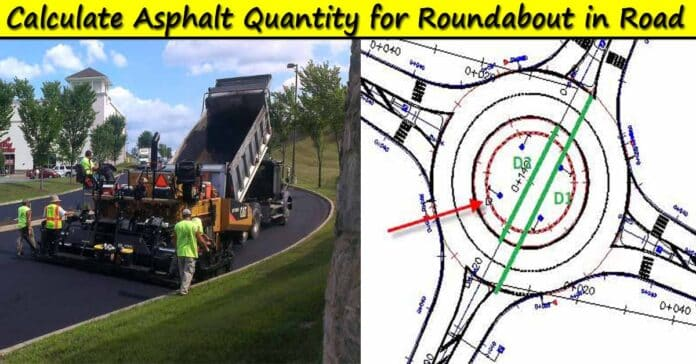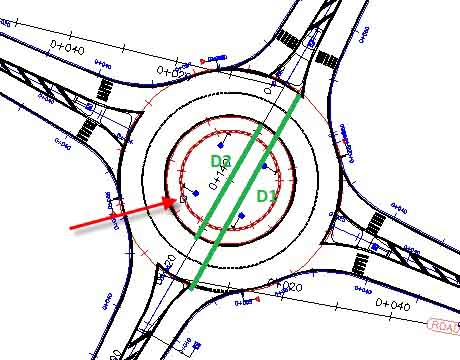How Calculate Asphalt Quantity for Round About in Road Construction, How to Calculate Quantity of Dump Trucks in Asphalt, How to Calculate Asphalt Quantity in Tons, What is the Formula to Calculate Asphalt

8# How Calculate Asphalt Quantity for Roundabout in Road Construction | How to Calculate Asphalt Quantity in Tons | Asphalt Compaction Factor

In this Article today we will talk about the How Calculate Asphalt Quantity for Round About in Road Construction | How to Calculate Quantity of Dump Trucks in Asphalt | How to Calculate Asphalt Quantity in Tons | What is the Formula to Calculate Asphalt | How to Measure Asphalt Quantity for Road | Asphalt Compaction Factor | Asphalt Base Course | Asphalt Wearing Course

roundabout (Traffic Circle) is a type of circular intersection or junction in which road traffic is permitted to flow in one direction around a central island, and priority is typically given to traffic already in the junction.

### How To Find The Quantity of Asphalt for Roundabout in Road:

#### Formula for Calculation of Asphalt in Road:

1. Find out the dimension (Outer Diameter and Inner Diameter)
2. Calculate the Area of Asphalt (Area of Asphalt and Area of Green Belt)
3. Calculate Volume of Asphalt = (Area * Thickness) m^3
4. Find out the density of Asphalt (145 lbs/cft to 2330 Kg/m3)
5. Calculate the quantity of Asphalt= ( Density* Volume)/1000
6. Use the Compaction Factor for AWC (1.28% – 1.30% in Asphalt) and for ABC (1.23% – 1.26% in Asphalt) How to Measure Asphalt Quantity for Road
7. Total Weight of Asphalt with Compaction Factor = Quantity of AWC x Compaction Factor
8. Calculate No of Dump Trucks = Total Weight of AWC Asphalt in Tones / Material in One Dump Truck Asphalt Base Course, Asphalt Wearing Course

#### Given Data (Example):Outer Diameter of Roundabout = D1 = 40 m

Inner Diameter of Roundabout = D2 = 10 m

Thickness of Asphalt Layer = H = 100 mm = 0.10 m

#### Quantity of Asphalt Wearing Course Calculation in Road:

Area of Asphalt Portion and Green Belt = A1 = Pi x D1 x D1 x 0.25

Area of Asphalt Portion and Green Belt = A1 = 3.142 x 40 x 40 x 0.25 = 1256.80 m2

Area of Green Belt = A2 = Pi x D2 x D2 x 0.25

Area of Green Belt = A2 = 3.142 x 10 x 10 x 0.25 = 78.55 m2

Net Area = Area of Asphalt Portion and Green Belt – Area of Green Belt

Net Area = A = A1 – A2 = 1256.80 – 78.55 = 1178.25 m2

Therefore, the Volumetric Quantity of Roundabout Section = V = Net Area x H

Volume of Roundabout = 1178.25 x 0.10 = 117.82 Cum

Here, we use a standard Density of Asphalt is 145 lbs/cft or 2330 Kg/m3 which may be somewhat different depending on the precise asphalt mixture.

Quantity of Roundabout = Volume of AWC x Density of Asphalt

Quantity of Roundabout = 117.82 x 2330 = 274520.60 Kg

Compaction Factor (1.28 to 1.30) = 1.26 %

Total Weight of Asphalt with Compaction Factor = Quantity of AWC x Compaction Factor

Total Weight of Roundabout Asphalt with Compaction Factor (Lose Material) = 274520.60 x 1.26 = 345895.956 Kg

Total Weight of Roundabout Asphalt in Tones = Total Weight of AWC Asphalt with Compaction Factor / 1000

Total Weight of Roundabout Asphalt in Tones = 345895.956 / 1000 = 345.89 Tons

#### How to Find No. of Trucks in Asphalt Quantity?

Material in One Dump Truck = 24 Ton

No of Dump Trucks = Total Weight of AWC Asphalt in Tones / Material in One Dump Truck

No of Dump Trucks = 345.89 / 24 = 14.41 Trucks (Lose Material)

## FAQ’s

### What is the formula to calculate asphalt?

Volume of Asphalt = L x W x H | Weight or Quantity of Asphalt = Volume of Asphalt x Density of Asphalt | Density of Asphalt = 145 lbs/cft to 2330 Kg/m3 | Quantity of Asphalt in Tons = Quantity of Asphalt / 1000

### How do I figure out how many dump trucks I need?

Measure (in feet) the length, width and height of the bed, multiply the three measurements together, and then divide by 24 to find out the capacity in cubic yards. After you make those calculations you'll be well on your way to transporting those much needed materials.

2.5 tonnes/m3

### How is earthwork quantity calculated?

The determination of earthwork quantities is based upon field cross- sections taken in a specified manner before and after excavation. Cross- sections are vertical profiles taken at right angles to the survey centerline. Every section is an area formed by the subgrade, the sideslopes, and the original ground surface.

### How many square meters is a ton of asphalt?

24 SqM | One ton of asphalt covers: 2” or 50.8 mm thick = 24 SqM.

## Conclusion:

1.Kabunga Nicodemus

i have loved this work.
Am doing Q.S and i want with roads.
so quantification of road materials is important to me

2.Tesfaye Nigatu

thank you so much for teaching us

• Thanks for the comments please visit more articles by clicking on “All Posts” tab of the Menu Bar

3.Talegin Siraj

I am interested and l love

• Thanks for the comments please visit more articles by clicking on “All Posts” tab of the Menu Bar

4.Eng Bilisuma

I am very interesting on this wabe

• Thanks for the comments please visit more articles by clicking on “All Posts” tab of the Menu Bar